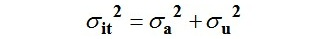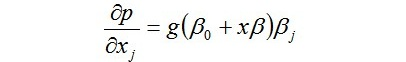Try it now!

USD
10

# International Comparison of Consumer Debts - Part 4

2285

## Chapter 2. Methodology Analysis

The methodology to output includes STATA software that creates an input and output and the graphical representations also included. The STATA converts the wave data to provide a horizontal and vertical comparison. These values after the calculations the data analyzed both in the graphical and manual method to provide the values.

/__ / ____/ / ____/

___/ / /___/ / /___/ 12.0 Copyright 1985-2011 STATA Corp LP

Statistics/Data Analysis   STATA Corp

4905 Lake way Drive

Special Edition     College Station, Texas 77845 USA

800-STATA-PC  http://www.stata.com

979-696-4600  stata@stata.com

979-696-4601 (fax)

Serial number: 93611859953

We have used a Panel Probit Regression technique.

Our data can be seen as a Panel data, data on variables measured for the same units (individuals here) across time (waves here).

If you have no clue about argumentative essay writing, the best way to ease your life is to use an online writing service. Purchase your essay from Pro-Papers and get a completely original paper for the best price.

### 2.1 Panel Data

Data sets that combine time series and cross sections are called longitudinal or panel data sets. If the same people or states or counties, sampled in the cross section, are then re-sampled at a different time we call this a longitudinal data set, which is a very valuable type of panel data set.

The following is a simple illustration of the basic framework in a panel regression:

yit0t1xit12xit2+ai+uit

here

yit is the value of y, the variable we are trying to explain, at year t,

xitj ,j=1,2,..k are time-varying individual characteristic of the i-th individual, e.g., income,

ai represents individual characteristics - those measurable chars. that do not change over time, e.g, gender, nationality etc, and also unobserved individual characteristics, e.g personality, intelligence - so-called unobserved heterogeneity .

uit is the regression error term, which contains all other unmeasured effects on Y. Often called the idiosyncratic error

It turns out that the key to estimating this model, is what we can assume about the ai terms.

#### 2.1.1 Fixed Effects Panel-data Model (FE) (individual-specific intercepts)

The effects of time-constant independent variables can not be consistently estimated because they are mixed within ai with unobserved individual characteristics, which are further correlated with the uit. ( This is also why a simple Pooled Regression, combining all the data points, ignoring the panel structure, will not work) So we use methods like mean-differencing , using dummy variables. etc.to control for them, and estimate the b' s

### 2.2 Random Effects Model (RE)

Here we think of ai as composed of a random part ai, varying only across individuals, but fixed over time-measurable individual characteristics that do not change over time, e.g, gender, nationality etc, and a unmeasurable element ni

i.e.,

ai =ai+ni

So the regression is now:

yit0t1xit12xit2+ai+ni +uit

We can think of the term ai+uit as the composite error.

eit=ai+uit

The key assumption in RE is:

ni is uncorrelated with each explanatory variable in all time periods. So we can proceed to combine it with the uit, and work with the .eit , which will have the usual desirable OLS residual characteristics , including Cov(Xit,eis)=0

Then we can also estimate the ai (effects of time-constant independent variables) as parameters.

This is the key difference between RE and FE estimators:

In FE, we assume that ai =ai+ni may be correlated with other explanatory variables, hence the ai cannot be estimated.

In RE, the unmeasurable part of ai is omitted and is part of the disturbance, while the measurable part can be estimated.

RE estimates are more efficient (or more precise) if the RE assumption is valid. On the other hand, if Cov(Xit,ai) is nonzero but the RE method is used, estimates of all parameters might be biased. This bias can be called heterogeneity bias.Since we are interesting in the effects of many of the time-constant independent variables, we use the RE method. But we have to recognize and estimate the extent of the heterogeneity bias. This is further explained below.

Recall the composite error.

eit = ai+uit

since a & u are uncorrelated, the variance of e is:A very significant fact is that the eit's are serially correlated, with the correlation being given by:The fact of this serial correlation of errors makes RE superior to a pooled regression, as we need to use GLS techniques to estimate the variance-covariance matrix, and use it for a weighted regression.

r or "rho" can be interpreted as the proportion of the total variance contributed by the panel-level (i.e. subject level) variance component

When rho is close to zero:

the panel-level variance component is unimportant. This means there is no significant individual heterogeneity, so we could estimate the parameters consistently by pooled regression- however, RE will be more efficient.

When rho is close to one:

The panel-level variance component is the most important element of error variance- there is high degree of significant individual heterogeneity. However in this case, RE estimates will be close to the FE estimates via the estimated variance-covariance matrix, and the adjustment mad in the RE technique. i.e., although there is significant unobserved heterogeneity, the bias in RE estimates is small.

### 2.3 Probit Regression

Further, since we have a binary dependent variable (y=owe_money=1 -> individual owes some kind of loan), we need to estimate the probability of y=1

So we do a Panel Probit regression of owe_money on a list of repressors (independent variables)

Binary variable represents observations obtained for a random variable with only two possible values. Typically, these two possible values are called a “success” and a “failure”. In our case, we can think of the case where owe_money=1 (i.e., individual is a borrower) as "success"

Thus, we are trying to explain the expected values of a variable y, which takes the value 1 if it's success, and 0 otherwise.

Let p be the probability of success. We assume that the expected value of y (which is equal to the probability of success) can be explained by some observed x variable

E(Y) = 1. p+0 (1- p)= p=p(x) = a + bx

This is called the Linear Probability Model (LPM).

However, this cannot be meaningfully estimated with an ordinary regression model (OLS):

• The first problem is that with this model, predicted probabilities can be less than 0 or greater than 1 !
• Further, it also Violates the usual distributional assumptions for OLS, since Y cannot be normally distributed (takes only 2 values!)

An alternative to estimating the LPM:

P(y = 1|x) = b0 + xb

is to model the probability of "success" as a non-linear function of x & b,

P(y = 1|x) = G(b0 + xb), where 0 < G(z) < 1.

When G(z) , so-called Link Function, is the standard normal cdf we call this a Probit Model. (When G(z) is the logistic function, we call this a Logit Model). Since this is now nonlinear in parameters, OLS is inappropriate and we must use maximum likelihood estimation.

However, interpreting the regression coefficients is now much more complicated than interpreting the LPM, since, where g(z) is dG/dz. Thus the effect of any xj depends not only on the sign & magnitude of bj, but also on the values of all the x's at the j-th observation. However, the sign of σp / σxj will be the same as the sign of bj

## Chapter 3. Empirical analysis of Consumer credit in Europe: a country comparison

### 3.1 Data

The data in the empirical analysis includes country, gender, age, education, higher or other education, family size, working status, residential status, financial status, and expenditures in food, investment in savings such as bonds, stock, and asset on land, insurance, and other policies. The number of cars, retirement age, and health status also analyzed. The empirical analysis states the comparison in the economic changes, in the various aspects in the country. The data for the survey from the four WAVE the WAVE four represent the latest survey and the WAVE three shows the different comparison in the credit of consumers so these two WAVE data analyzed. The depended values mostly the people of the country.

We decided to study 11 European countries as in the Haliasos study ("Differences in Portfolios across Countries: Economic Environment versus Household Characteristics", Christelis, Georgarakos & Haliassos) Sweden, Denmark, Germany, Netherlands, Belgium, France, Switzerland, Austria, Italy, Spain, and Greece.

### 3.2 Data Sources

SHARE & EASYSHARE

We started with the SHARE (Survey of Health, Ageing and Retirement in Europe.. Wave 1 (2004), Wave 2 (2006-07) & Wave 4 (2010-11)) datasets for Waves 1, 2 & 4 (years 2004-11). Due to the large number of missing responses (Table 2 in the appendix gives some details of the number of available & missing data for the SHARE variables of interest to us), especially for economic & financial variables, we supplemented SHARE with the Easyshare data from the same source.

Table 1 in the Appendix lists variables used, with sources & original names:

Table 2 in Appendix details the availability for various variables – note the overwhelmingly large no. of missing for financial variables. This is also the reason we could not estimate any model for levels of credit, but only the probability of borrowing.

### 3.3 Dependent variable

In the AS (Assets) module the following question relate to credit (money owed):

AS054_ OWE MONEY

"Looking at card 34, which of these types of debts do you [or] [your] [husband/wife/partner] currently have, if any?"

None of the questions are directly representative of consumer credit. But since this is what the dataset offers, we have to make the best use of it. Accordingly, we have assumed that we can approximate our target variable by combining all the categories.

Further, as a matter of convenience in programming, we actually use the information on the answers to the residual category:

as054dno "owe money: none of these"

Those who answer 'No" to this question are the ones who have some sort of loan. So we have constructed a new variable,

owe_money=1- as054dno

to represent individuals who have some type of loan

Do you want to have the best personal statement in the class but don’t know how to compose it? Find out the most professional personal statement writers at Pro-Papers to solve your problem.

#### 3.3.4 Regressors (Explanatory/Independent Variables)

Variable

Notes

country

Country code

female

Gender indicator (0-Male, 1 female)

marital_status

age

hhsize

education_level

Standard categories from Easyshare (explained later)

employment_status

income_pct

Income Percentile

amount_bank_account*

earning_before_taxes*

total_income_other_hh_members*

*Dropped in final regression due to overwhelmingly large no. of missing (see Table 2 in Appendix)Leave a Reply